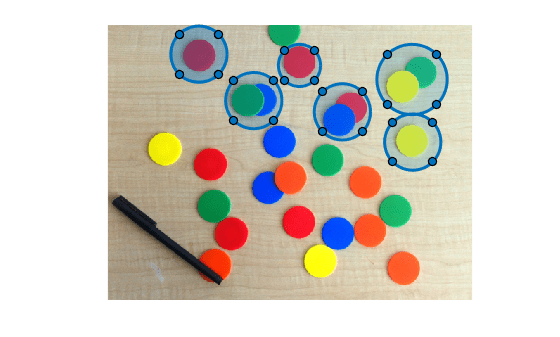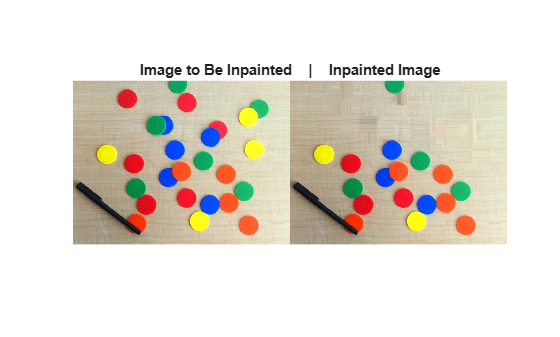# inpaintCoherent

Restore specific image regions using coherence transport based image inpainting

Since R2019a

## Syntax

``J = inpaintCoherent(I,mask)``
``J = inpaintCoherent(I,mask,Name,Value)``

## Description

example

````J = inpaintCoherent(I,mask)` restores specific regions in the input image using the coherence transport based inpainting method. `mask` is a logical image that denotes the target regions in the image to be filled through inpainting.```

example

````J = inpaintCoherent(I,mask,Name,Value)` specifies additional inpainting options using one or more name-value arguments.```

## Examples

collapse all

Read an image to be inpainted into the workspace. This image contains text overlays to be removed.

`I = imread('overlayimage.png');`

Read the mask image into the workspace. This mask image contains the overlayed text regions present in the image to be inpainted.

`mask = imread('text.png');`

Display the image to be inpainted and its corresponding mask image.

```montage({I,mask}); title(['Image to Be Inpainted',' | ','Mask for Inpainting'])```Inpaint the original image by removing the text overlays.

`J = inpaintCoherent(I,mask);`

Display the original image and the inpainted image.

```montage({I,J}); title(['Image to Be Inpainted',' | ','Inpainted Image'])```Read an image to be inpainted into the workspace.

`I = imread('coloredChips.png');`

Display the image.

```figure imshow(I,[])```

Use the `drawcircle` function to select a circular region of interest (ROI) for inpainting. Use the `Center` and `Radius` name-value pairs to specify the location of an ROI.

`h = drawcircle('Center',[130,42],'Radius',40);`Select Multiple ROIs for Inpainting

You can also select multiple ROIs iteratively.

Set the number of regions to be inpainted to 6.

`numRegion = 6;`

Specify the center and radii for each region.

```roiCenter = [130 42;433 78;208 108;334 124;434 167;273 58]; roiRadius = [40 50 40 40 40 30];```

Select multiple circular ROIs iteratively by specifying the `drawcircle` `Center` and `Radius` name-value pairs.

```roi = cell([numRegion,1]); for i = 1:numRegion c = roiCenter(i,:); r = roiRadius(i); h = drawcircle('Center',c,'Radius',r); roi{i} = h; end```Use the `createMask` function to generate a mask from the selected ROIs.

```mask = zeros(size(I,1),size(I,2)); for i = 1:numRegion newmask = createMask(roi{i}); mask = xor(mask,newmask); end```

Display the image to be inpainted and its corresponding mask image.

```montage({I,mask}); title(['Image to Be Inpainted',' | ','Mask for Inpainting'])```Remove objects in the ROIs through inpainting. Specify a standard deviation of 0.5 and an inpainting radius of 1.

`J = inpaintCoherent(I,mask,'SmoothingFactor',0.5,'Radius',1);`

Display the original image and the inpainted image.

```montage({I,J}); title(['Image to Be Inpainted',' | ','Inpainted Image']);```## Input Arguments

collapse all

Image to inpaint, specified as a grayscale image of size m-by-n or an RGB color image of size m-by-n-by-3.

Data Types: `single` | `double` | `int8` | `int16` | `int32` | `uint8` | `uint16` | `uint32`

Spatial mask of target regions, specified as a 2-D binary image of size m-by-n, where m and n are the dimensions of input image `I`. The nonzero pixels in `mask` constitute the target regions to be filled through inpainting.

Note

Data Types: `logical`

### Name-Value Arguments

Specify optional pairs of arguments as `Name1=Value1,...,NameN=ValueN`, where `Name` is the argument name and `Value` is the corresponding value. Name-value arguments must appear after other arguments, but the order of the pairs does not matter.

Before R2021a, use commas to separate each name and value, and enclose `Name` in quotes.

Example: `J = inpaintCoherent(I,mask,'Radius',7)`

Standard deviation of Gaussian filter, specified as a positive number. This value is used to compute the scales of the Gaussian filters while estimating the coherence direction.

Inpainting radius, specified as a positive integer. The inpainting radius denotes the radius of the circular neighborhood region centered on the pixel to be inpainted.

## Output Arguments

collapse all

Inpainted image, returned as a grayscale image or RGB color image of the same size and data type as input image `I`.

## Tips

• The inpainting results depend on the name-value pair specification. You can modify the values of `Radius` and `SmoothingFactor` for varied results.

• Each ROI in the binary mask image must be sufficiently large to enclose the corresponding region in the image to be inpainted.

## Algorithms

The coherence transport based inpainting method is a pixel-based approach for removing objects and filling regions in images . Inpainting is performed inwards starting from the boundary pixels of the target region. The inpainting value for a pixel is estimated from its coherent neighboring pixels with known values. The steps involved are summarized as follows:

1. Identify target regions from the input image to be filled or inpainted. Generate a binary mask of the size same as the input image. The nonzero pixels in the mask image must contain the target regions to be inpainted.

The order in which the pixels in the target region are inpainted is calculated from their Euclidean distance to the boundary of the target region.

2. The inpainting value for a pixel in the target region is the weighted average of known pixel values within its inpainting radius. The known pixels along the coherence direction are assigned higher weight value than the incoherent neighboring pixels. The coherence direction is estimated by using a structure tensor.

 F. Bornemann and T. März. "Fast Image Inpainting Based on Coherence Transport." Journal of Mathematical Imaging and Vision. Vol. 28, 2007, pp. 259–278.